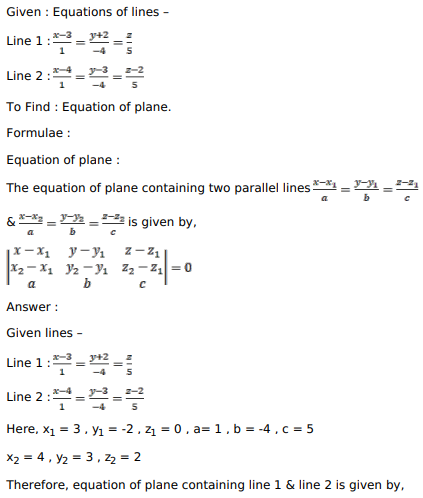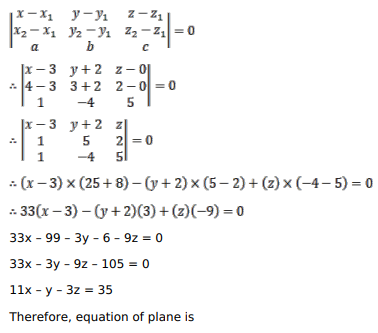# Find the equation of the plane which contains two parallel lines givenQuestion:

Find the equation of the plane which contains two parallel lines given by $\frac{x-3}{1}=\frac{y-2}{-4}=\frac{z}{5}$ and $\frac{x-4}{1}=\frac{y-3}{-4}=\frac{z-2}{5}$.

Solution: